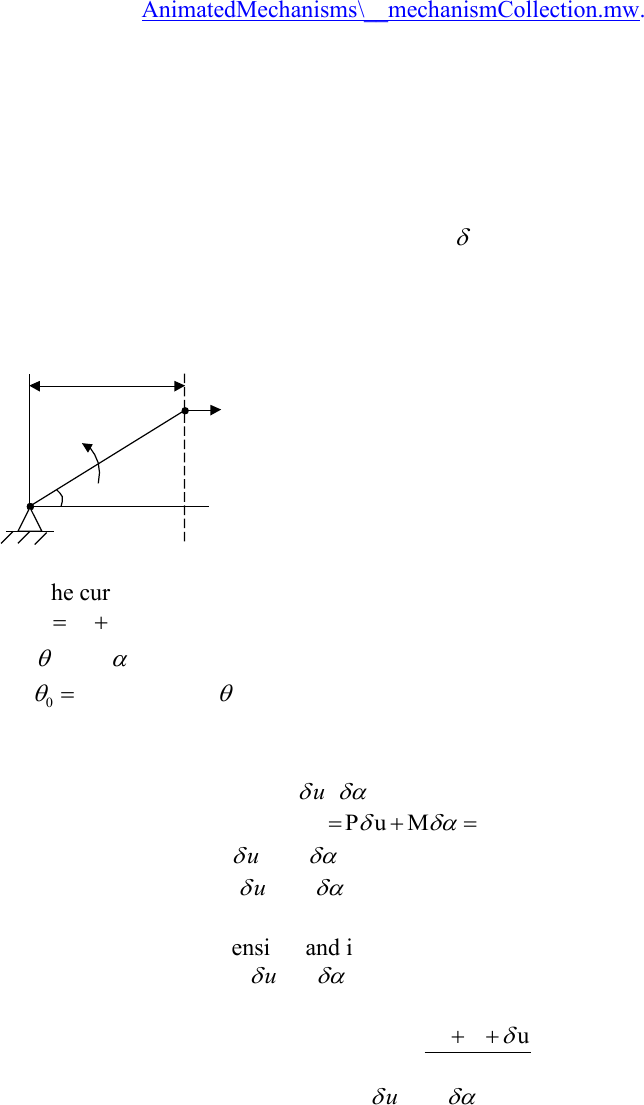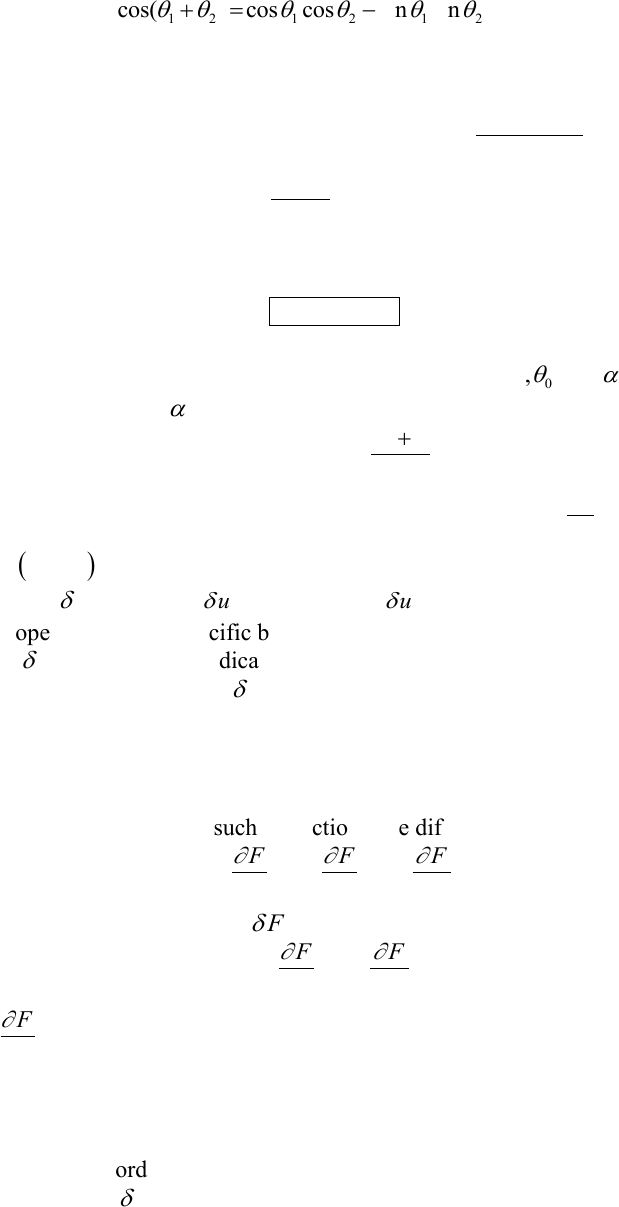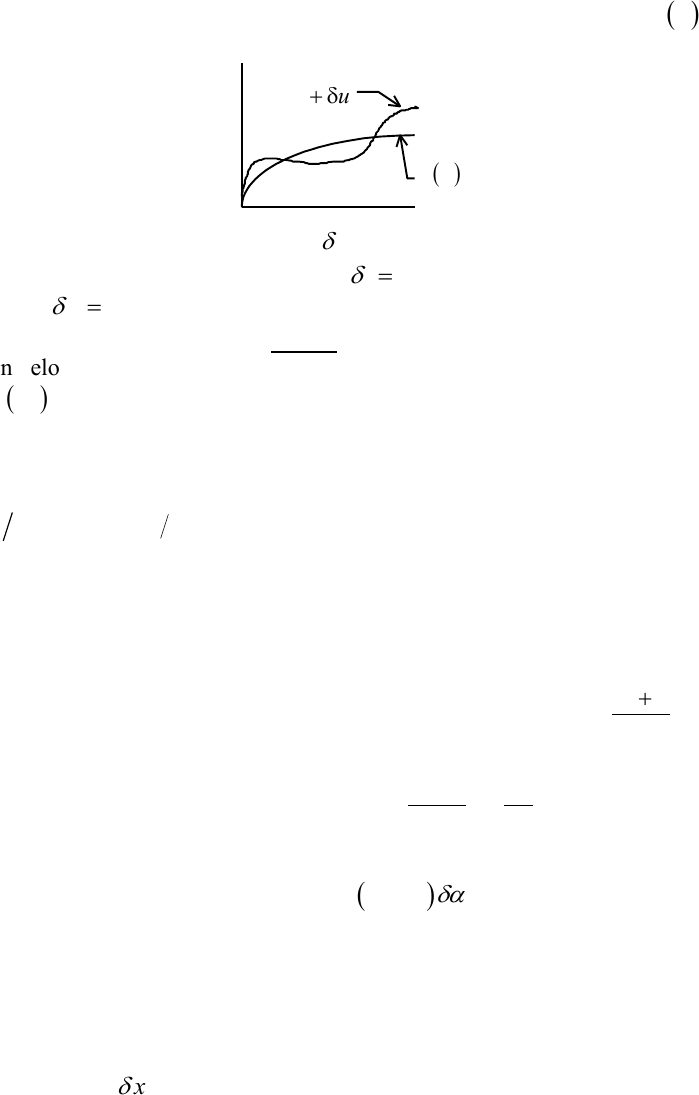Study Guides (390,000)
US (220,000)
TAMU (3,000)
AERO (10)
Final

# AERO 306 Lecture 2: 2_Dealing_with_complex_kinematicsExam

Department
Aerospace Engineering
Course Code
AERO 306
Professor
John Whitcomb
Study Guide
Final

This preview shows pages 1-2. to view the full 8 pages of the document.C:\Users\cloudconvert\server\files\119\172\7\e600a07f-34c2-41c0-9379-
fe795617d10e\7d65bb12cc93584b7fc0ec4f3649ac.doc
p. 1 of 8
Dealing with Complex Kinematics
When the relationships between various displacements are not very simple, it is useful to
get some help from your calculus background. Several examples are illustrated in this
Maple worksheet: AnimatedMechanisms\__mechanismCollection.mw.
The next section introduces the “variational operator”, which is closely related to the
differential operator that you saw in your first calculus course. You will find that using
the variational operator greatly simplifies many problems.
Pre-calculus approach (the hard way)
When the kinematics are not trivial, it is useful to understand something called the
“variational operator”. Up until now, we have used
as a label to indicate a virtual
displacement. It actually means more than that… it is referred to as the variational
operator. Before explaining what this new operator is, let’s first solve the following
problem without using it. This example will help motivate the need for the variational
operator.
P, u
a
M
Inextensible bar of
length L
Suppose the current equilibrium state is as shown
0
0
a a u
=+
=+
0
0
where initial valueof u=translation in direction of P
a initial valueof a α=rotation in direction of

=
=
Now impose virtual displacements
,u
VW P u M 0

= + =
This is the virtual work, but
u and
are not independent. To proceed further we must
find the relationship between
u and
. If we assume we do not know about calculus,
we can proceed as follows:
If we assume the bar is inextensible and it's length = L, then simple trigonometry will
give us a relationship between
.
( )
0
0
a u u
cos L
 
++
+ + =
This can be simplified by recognizing that
u and
are infinitesimal.

Only pages 1-2 are available for preview. Some parts have been intentionally blurred.C:\Users\cloudconvert\server\files\119\172\7\e600a07f-34c2-41c0-9379-
fe795617d10e\7d65bb12cc93584b7fc0ec4f3649ac.doc
p. 2 of 8
1 2 1 2 1 2
0 0 0
Recall the formula: cos( ) cos cos sin sin
cos( ) cos( )cos( ) sin( )sin( )
But is extremelysmall sin cos 1and
        
   
+ =
+ + = + +
 = =
0
0 0 0
0
0
0
a u u
cos( ) cos( ) sin( ) L
au
but cos( ) L
sin( )
Therefore
uL
       

 
++
+ + = + + =
+
+=
= − +
Now substitute this relationship into the expression for VW and solve to obtain
M PLsin
=
There is an easier way. Regardless of the particular values of
00
,,a and

, the
relationship between u and
is given by
( )
0
0
au
cos L

+
+=
Take the differential of both sides, and we obtain:
( )
0
du
sin L
d
 
+ =
. Hence,
( )
0
sindu L d
 
= − +
, which is very close to what we obtained above. The difference
, e.g.
.du vs u
. Both
du and u
refer to shall changes in "u". The
differential operator "d" has specific behavior that you have studied before in math
classes. The
was just used to indicate some small change in a displacement… up until
now. Now we are going to define
to be the variational operator and it will have
properties that are very similar to the differential operator, as described next.
Variational Operator
Suppose we have a function F = F (x,u,u’), where u(x) is a displacement field. The strain
energy density is an example of such a function. The differential of the function is
F F F
dF d x d u d u
x u u
 
= + +
In contrast, the variation of F… i.e.
F
is
FF
F u u
uu

 

=+
The term
Fdx
x
does not appear in the variation of F. The differential is meant to
describe changes in the function due to all possible effects: changes in position x, u, and
u'. The variation only describes changes that are related to displacement. In this class a
simple rule will suffice to be able to take a variation of a function: Take the differential of
the function, set all coordinate differentials (e.g. dx and dy) to zero, and then simply
replace the "d" with a
. The following simply illustrate the properties of the variational
operator. No new properties are stated.

Unlock to view full version

Only pages 1-2 are available for preview. Some parts have been intentionally blurred.C:\Users\cloudconvert\server\files\119\172\7\e600a07f-34c2-41c0-9379-
fe795617d10e\7d65bb12cc93584b7fc0ec4f3649ac.doc
p. 3 of 8
Suppose u=u(x). We will define
( )
to be the variation (small change) of u xu
(see
plot below).
u u+
( )
u x
Suppose ci = a list of constants. Then
ci
= changes in the values of those constants.
At points where u(x) is fixed (specified),
u=0
. Similarly, if the constant c2 is
specified,
c20=
The variational operator behaves almost exactly like the differential operator, as
shown below.
( )
( )
( )
( ) ( )
1nn
bb
aa
uv u v v u
u v u v
u n u u
d dx u du dx
udx udx
 
 



=+
+ = +
=
=
=

Example
In the leaning bar problem earlier, we had the relationship
( )
0
0
au
cos L

+
+=
.
According to the rules above
( )
( )
( )
0
00
00
au
cos sin L
The variations of , a , and L are all zero, since all are fixed values.
u
and L
    
+

+ = + =


Hence, we obtain the relationship
( )
0
sinuL
=− +
.
In this case, the "thing" being varied was a single number. Suppose the displacement of a
beam was being approximated by the polynomial function
23
()v x a bx cx dx= + + +
,
where a, b, c, and d are all constants which are not specified, but will be determined
somehow. The variation of v(x) is
23
()v x a x b x c x d
 
= + + +
Note that there is no
x
term!
Later in the course we will learn how we can assume such a function for the transverse
displacement of a beam and determine the constants a, b, c, and d in a systematic manner.